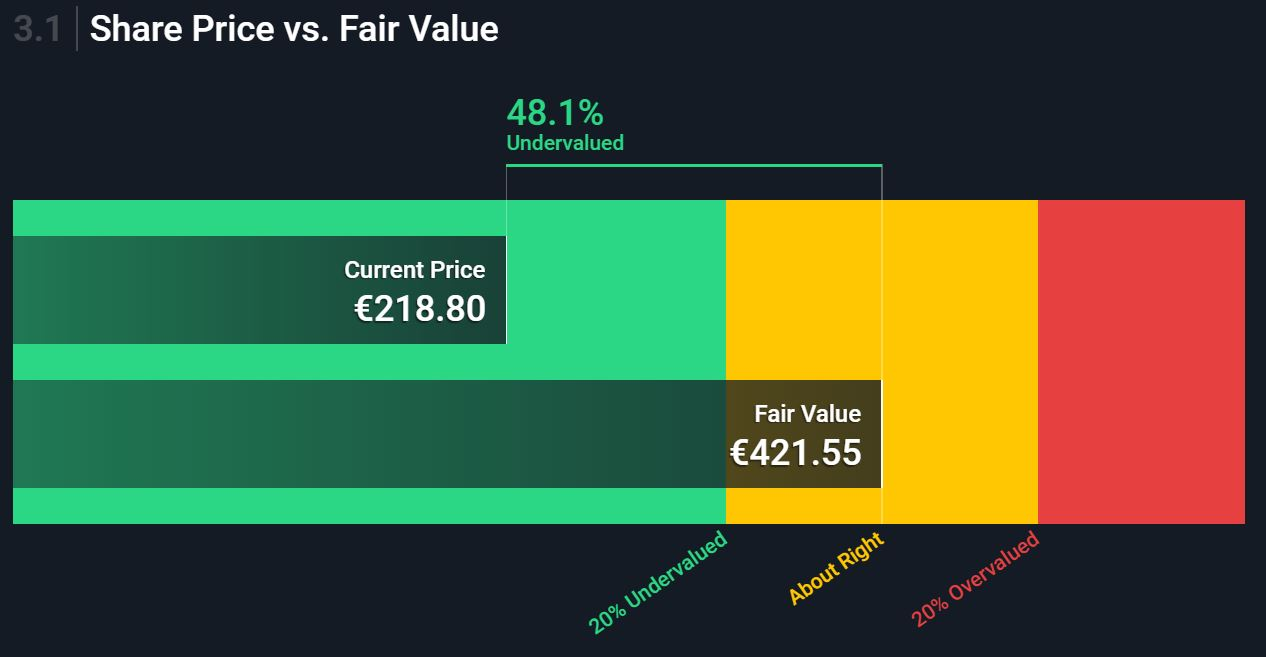# Simplywall.st Share Price vs. Fair Value

SimplyWall.st have an indicator called: Share Price vs. Fair Value

I love it, it allows me to simplify so many things. It’s shown as (stock used Allianz (ALV)):The important thing is how the Fair value is calculated.

Below are the data sources, inputs and calculation used to determine the intrinsic value for Allianz.

XTRA:ALV Discounted Cash Flow Data Sources
Data Point Source Value
Valuation Model Excess Returns Model
Stable EPS Weighted future Return on Equity estimates from 16 analysts.
= Stable Book Value * Return on Equity
= €183.66 * 0.1%
€23.33
Book Value of Equity per Share Weighted future Book Value estimates from 10 analysts. €183.66
Discount Rate (Cost of Equity) See below 5.7%
Perpetual Growth Rate 10-Year DE Government Bond Rate 0.2%

An important part of a discounted cash flow is the discount rate, below we explain how it has been calculated.

Calculation of Discount Rate/ Cost of Equity for XTRA:ALV
Data Point Calculation/ Source Result
Risk-Free Rate 10-Year DE Govt Bond Rate 0.2%
Equity Risk Premium S&P Global 6.0%
Insurance Unlevered Beta Simply Wall St/ S&P Global 0.67
Re-levered Beta = Unlevered beta (1 + (1- tax rate) (Debt/Equity))
= 0.667 (1 + (1 – 30.0%) (43.19%))
0.912
Levered Beta Levered Beta limited to 0.8 to 2.0
(practical range for a stable firm)
0.91
Discount Rate/ Cost of Equity = Cost of Equity = Risk Free Rate + (Levered Beta * Equity Risk Premium)
= 0.23% + (0.912 * 5.96%)
5.66%

Discounted Cash Flow Calculation for XTRA:ALV using Excess Returns Model Model

The calculations below outline how an intrinsic value for Allianz is arrived at using the Excess Return Model. This approach is used for finance firms where free cash flow is difficult to estimate.

In the Excess Return Model the value of a firm can be written as the sum of capital invested currently in the firm and the present value of excess returns that the firm expects to make in the future.

The model is sensitive to the Return on Equity of the company versus the Cost of Equity, how these are calculated is detailed below the main calculation.

Note the calculations below are per share.

XTRA:ALV Value of Excess Returns
Calculation Result
Excess Returns = (Stable Return on equity – Cost of equity) x (Book Value of Equity per share)
= (0.1% – 5.66%) x €183.66
€12.93
Terminal Value of Excess Returns = Excess Returns / (Cost of Equity – Expected Growth Rate)
= €12.93 / (5.66% – 0.23%)
€237.89
Value of Equity = Book Value per share + Terminal Value of Excess Returns
EUR183.66 + €237.89
€421.55
XTRA:ALV Discount to Share Price
Calculation Result
Value per share (EUR) From above. €421.55
Current discount Discount to share price of €218.8
= -1 x (€218.8 – €421.55) / €421.55
48.1%